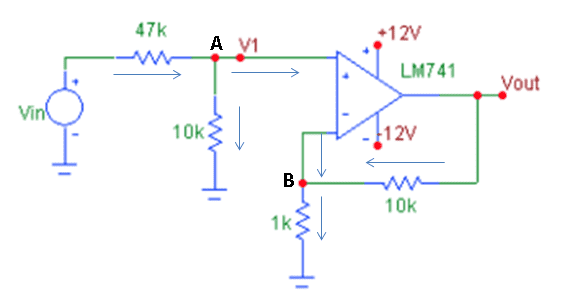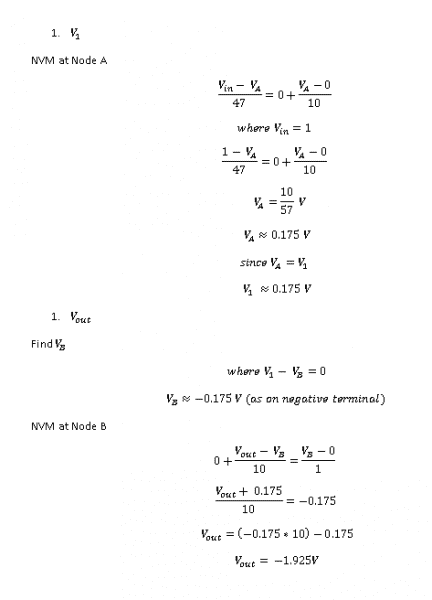# Non Inverting Op-Amp Circuit Analysis

• Engineering
• cavalieregi

## Homework Statement

For an input potential (Vin ) which is a 1V (peak) sinusoidal source. Calculate V1 and Vout## Homework Equations

- The laws for an op-amp
- NVM

## The Attempt at a Solution

Here is my attempt not sure if correct.#### Attachments

VB = VA otherwise the OP-AMP is not operating as an amplifier. Fix this and you should be right.

This is a non-inverting amplifier, the input being applied to the (+) input.

VB = VA otherwise the OP-AMP is not operating as an amplifier. Fix this and you should be right.

This is a non-inverting amplifier, the input being applied to the (+) input.
Cool, just wondering why it is a negative terminal ?

Cool, just wondering why it is a negative terminal ?
Why what is a negative terminal?

Why what is a negative terminal?
On diagram it has (-) for non inverting input.

On diagram it has (-) for non inverting input.
(-) is the inverting input of the OP-AMP, used here for negative feedback of some output to reduce the gain from its high open-loop value. The circuit's voltage input is applied to the (+) non-inverting input of the OP-AMP.

•cavalieregi
in addition to Nascent's points, you did some math solving for Vb incorrectly.
you say V1-Vb=0

so that means V1=Vb
why invert Vb?

Dont worry so much about what terminal is negative and so forth assuming this is an ideal amp.
To master op amps just stick to nodal analysis.
In this case, V1=A=B. They are all the same voltage.
We know that no current enters the op amp thru the + and - terminals.
Therefore we can easily find the voltage at A or V1 by using a simple voltage divdier. Vin X (10K/57K) = A
Now you know the voltage at B since the + and - terminals are equal in ideal amps.
Now use nodal analysis, (B / 1k) + (B - Vout)/ 10K = 0.
Solve and you are done.

•Dhruv Dhawan and cavalieregi